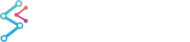﻿ Moving Average Filter | WPF Chart DocumentationSciChart WPF 2D Charts > Filters API > Moving Average Filter
Moving Average Filter

The Moving Average filter performs an N-period moving average, resulting in a smoothed waveform derived from your data, where N is passed to the function .ToMovingAverage().

To calculate a moving average and apply to a chart, use the following code.

Offset Filter
Copy Code
```using SciChart.Charting.Model.Filters; // Required for extension method .ToMovingAverage()

var dataSeries = new XyDataSeries<double,double>(); // Original Data
dataSeries.Append(0,1);
dataSeries.Append(2,2);

int period = 10;
var maDataSeries = dataSeries.ToMovingAverage(period); // Compute the moving average.

var lineRenderableSeries = new FastLineRenderableSeries()
{
DataSeries = maDataSeries, // Apply the Moving Average Data to a Line Series
}
```